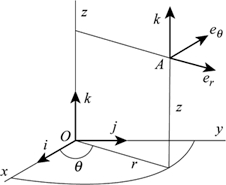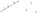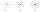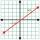# Parallel and orthogonal

I need math help in this problem: a=(-5, 5 3) b=(-2,-4,-5) (they are vectors)
Decompose the vector b into b=v+w where v is parallel to a and w is orthogonal to a, find v and w

Result

v1 =  2.119
v2 =  -2.119
v3 =  -1.271
w1 =  -4.119
w2 =  -1.881
w3 =  -3.729

#### Solution:

$a = (-5, 5, 3) \ \\ b = (-2,-4,-5) \ \\ \ \\ b = v+w \ \\ v \parallel a = > v = k a \ \\ \ \\ w \perp a = > w.a = 0 \ \\ \ \\ \ \\ -2 = v_{ 1 }+w_{ 1 } \ \\ -4 = v_{ 2 }+w_{ 2 } \ \\ -5 = v_{ 3 }+w_{ 3 } \ \\ v_{ 1 } = -5 \cdot \ k \ \\ = 2.119 \ \\ v_{ 2 } = 5 \cdot \ k \ \\ v_{ 3 } = 3 \cdot \ k \ \\ w_{ 1 } \cdot \ (-5)+w_{ 2 } \cdot \ 5 + w_{ 3 } \cdot \ 3 = 0 \ \\ \ \\ v_{ 1 }+w_{ 1 } = -2 \ \\ v_{ 2 }+w_{ 2 } = -4 \ \\ v_{ 3 }+w_{ 3 } = -5 \ \\ 5k+v_{ 1 } = 0 \ \\ 5k-v_{ 2 } = 0 \ \\ 3k-v_{ 3 } = 0 \ \\ 5w_{ 1 }-5w_{ 2 }-3w_{ 3 } = 0 \ \\ \ \\ k = \dfrac{ -25 }{ 59 } \doteq -0.423729 \ \\ v_{ 1 } = \dfrac{ 125 }{ 59 } \doteq 2.118644 \ \\ v_{ 2 } = \dfrac{ -125 }{ 59 } \doteq -2.118644 \ \\ v_{ 3 } = \dfrac{ -75 }{ 59 } \doteq -1.271186 \ \\ w_{ 1 } = \dfrac{ -243 }{ 59 } \doteq -4.118644 \ \\ w_{ 2 } = \dfrac{ -111 }{ 59 } \doteq -1.881356 \ \\ w_{ 3 } = \dfrac{ -220 }{ 59 } \doteq -3.728814 \ \\$
$v_{ 2 } = (-2.1186) = - \dfrac{ 125 }{ 59 } \doteq -2.1186 = -2.119$
$v_{ 3 } = (-1.2712) = - \dfrac{ 75 }{ 59 } \doteq -1.2712 = -1.271$
$w_{ 1 } = (-4.1186) = - \dfrac{ 243 }{ 59 } \doteq -4.1186 = -4.119$
$w_{ 2 } = (-1.8814) = - \dfrac{ 111 }{ 59 } \doteq -1.8814 = -1.881$
$w_{ 3 } = (-3.7288) = - \dfrac{ 220 }{ 59 } \doteq -3.7288 = -3.729$Our examples were largely sent or created by pupils and students themselves. Therefore, we would be pleased if you could send us any errors you found, spelling mistakes, or rephasing the example. Thank you!

Leave us a comment of this math problem and its solution (i.e. if it is still somewhat unclear...):Be the first to comment!Tips to related online calculators
Two vectors given by its magnitudes and by included angle can be added by our vector sum calculator.
Do you have a system of equations and looking for calculator system of linear equations?

## Next similar math problems:

1. Find the 10Find the value of t if 2tx+5y-6=0 and 5x-4y+8=0 are perpendicular, parallel, what angle does each of the lines make with the x-axis, find the angle between the lines?
2. Three points 2The three points A(3, 8), B(6, 2) and C(10, 2). The point D is such that the line DA is perpendicular to AB and DC is parallel to AB. Calculate the coordinates of D.
3. Set of coordinatesConsider the following ordered pairs that represent a relation. {(–4, –7), (0, 6), (5, –3), (5, 2)} What can be concluded of the domain and range for this relation?
4. Two peopleTwo straight lines cross at right angles. Two people start simultaneously at the point of intersection. John walking at the rate of 4 kph in one road, Jenelyn walking at the rate of 8 kph on the other road. How long will it take for them to be 20√5 km apa
5. Angle between vectorsFind the angle between the given vectors to the nearest tenth of a degree. u = (-22, 11) and v = (16, 20)
6. Parametric formCalculate the distance of point A [2,1] from the line p: X = -1 + 3 t Y = 5-4 t Line p has a parametric form of the line equation. ..
7. Coordinates of square verticesThe ABCD square has the center S [−3, −2] and the vertex A [1, −3]. Find the coordinates of the other vertices of the square.
8. Find the 5Find the equation with center at (1,20) which touches the line 8x+5y-19=0
9. Points collinearShow that the point A(-1,3), B(3,2), C(11,0) are col-linear.
10. CuboidsTwo separate cuboids with different orientation in space. Determine the angle between them, knowing the direction cosine matrix for each separate cuboid. u1=(0.62955056, 0.094432584, 0.77119944) u2=(0.14484653, 0.9208101, 0.36211633)
11. TriangleTriangle KLM is given by plane coordinates of vertices: K[11, -10] L[10, 12] M[1, 3]. Calculate its area and its interior angles.
12. Right triangle from axesA line segment has its ends on the coordinate axes and forms with them a triangle of area equal to 36 square units. The segment passes through the point ( 5,2). What is the slope of the line segment?
13. Right angled triangle 2LMN is a right angled triangle with vertices at L(1,3), M(3,5) and N(6,n). Given angle LMN is 90° find n
14. LineStraight line passing through points A [-3; 22] and B [33; -2]. Determine the total number of points of the line which both coordinates are positive integers.
15. Slope formFind the equation of a line given the point X(8, 1) and slope -2.8. Arrange your answer in the form y = ax + b, where a, b are the constants.
16. SlopeCalculate the slope of a line that intersects points (-84,41) and (-76,-32).
17. PerpendicularWhat is the slope of the perpendicular bisector of line segment AB if A[9,9] and B[9,-2]?